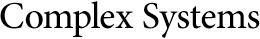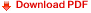## An Agent-Based Model of COVID-19Christopher Wolfram
Wolfram Research, Inc.
Many simple models of disease spread assume a homogeneous population (or population groups) with a uniform basic reproduction number (${R}_{0}$). The goal here is to develop and analyze an agent-based model of disease that models: (1) variability of interaction rates between agents; and (2) the structure of the in-person contact network.